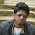### Citrix Written Test Questions-2

11. Find the slope of the line joining the points (0,2) and (3, -2)
a. -4/3
b. ¾
c. -3/4
d. 4/3

12. Solve for x in x^3+3x^2+5x+9=6
a. -1
b. 1
c. -2
d. -3

13. 73 to the base x is equivalent to 51 to the base y. which of the following may be the possible values of x and y
a. 10, 12
b. 8, 16
c. 9, 13
d. 8, 12

14. Which of the following is independent of the operating system and machine architecture
c. compiler
d. debugger

15. Which of the following restricts a process to the memory allocated to it
a. stack pointers
b. memory allocation hardware
c. kernel
d. none of these

16. When a user logs out, what happens to the processes created by him?
a. they’ll be killed
b. aborted
c. hang
d. none of these

17. What is the output of
Main()
{
Struct node {
Int a;
Int b;
Int c;
};
Struct node a = {2,4,5};
Struct node *st;
St = &a;
Printf(“%d”, *(int *)st);
}
a. 2
b. 3
c. 7
d. 11

18. What is the output of
Struct outer{
Struct inner{
Int a = 10;
}
Int b = 5;
}
Main()
{
Struct inner new;
Printf(“%d”, new.a)
}
a. It will give a compilation error
b. 10
c. 5
d. 15

19. Thrashing is solved by
a. increasing cpu bound processes
b. reducing the degree of multi-programming
c. increasing user processes
d. none of these

20. If t` is the reverse of t then what is the reverse of trs
a. s’r’t’
b. srt
c. t’r’s’
d. t’rs’

1.hi bapineedu.....
nice work ....
do me a favour ....could u plz post the interview questions asked by citrix......i really need it .....as soon as possible ....
thank u

2.3.4.